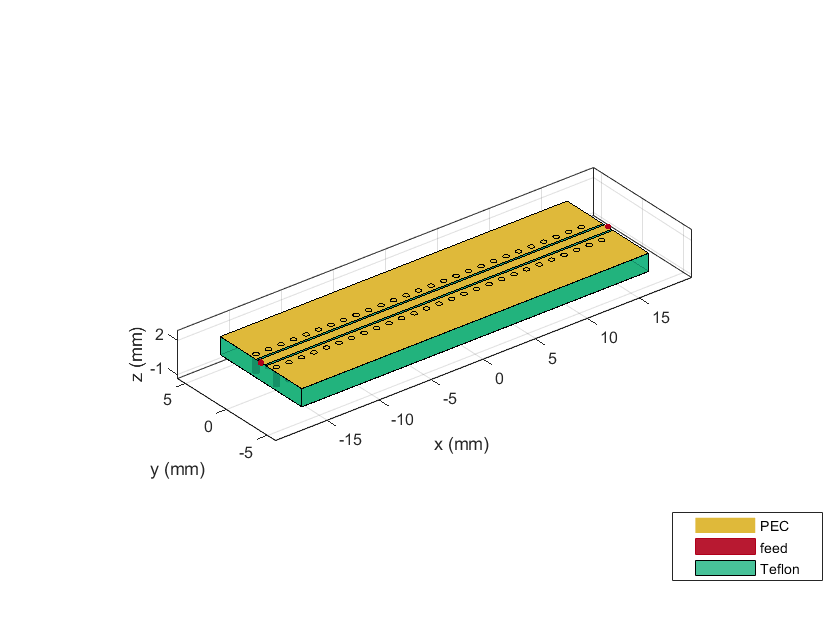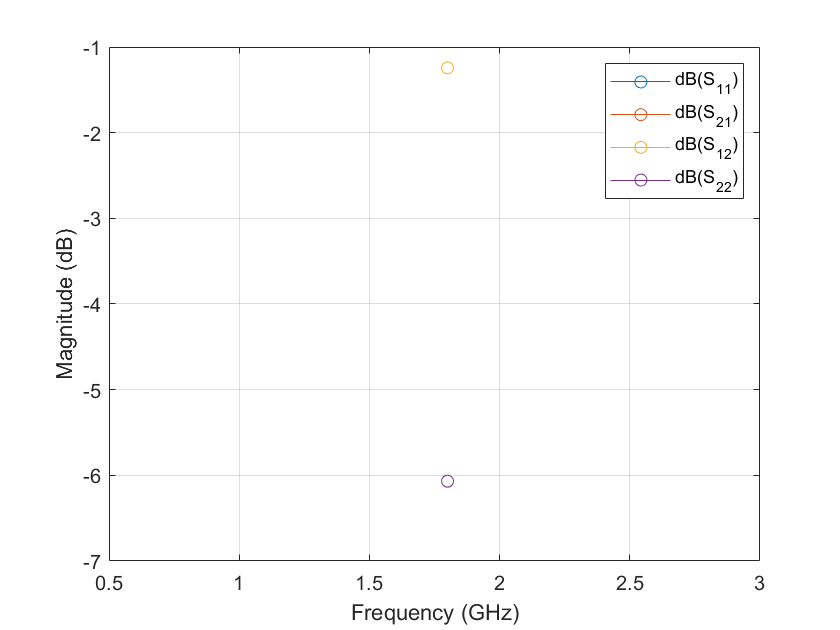# design

Design coplanar waveguide transmission line around particular frequency

Since R2021b

## Syntax

``waveguide = design(cpw,frequency)``
``waveguide = design(___,Name=Value)``

## Description

````waveguide = design(cpw,frequency)` designs an coplanar waveguide line around the specified frequency.```

example

````waveguide = design(___,Name=Value)` designs a coplanar waveguide with additional options specified using name-value arguments. NotePCB components designed using the `design` function operates around the specified frequency with a 10-15% tolerance. ```

## Examples

collapse all

Design a lambda/4 coplanar waveguide at 1.8 GHz with a characteristic impedance of 75 ohms.

```cpw = design(coplanarWaveguide, 1.8e9,Z0=75,LineLength=0.25); figure; show(cpw);```Plot the s-parameters of the waveguide at 1.8 GHz.

```spar = sparameters(cpw,1.8e9); rfplot(spar)```## Input Arguments

collapse all

Coplanar waveguide transmission line, specified as a `coplanarWaveguide` object.

Example: ```waveguide = coplanarWaveguide; waveguide = design(waveguide,2e9)``` designs a coplanar waveguide around a frequency of 2 GHz.

Design frequency of the transmission line, specified as a real positive scalar in hertz.

Example: `3e9`

Data Types: `double`

### Name-Value Arguments

Specify optional pairs of arguments as `Name1=Value1,...,NameN=ValueN`, where `Name` is the argument name and `Value` is the corresponding value. Name-value arguments must appear after other arguments, but the order of the pairs does not matter.

Example: `Z0=75`

Characteristic impedance of the line, specified as a positive scalar in ohms.

Data Types: `double`

Length of the coplanar waveguide, specified as a positive scalar in terms of Lambda.

Data Types: `double`

## Output Arguments

collapse all

Coplanar waveguide operating around the specified reference frequency, returned as a `coplanarWaveguide` object.

## Version History

Introduced in R2021b